### Maths MCQ Class IX Ch-14 | Statistics

Mathematics  MCQ | Class 09 | Chapter 14 STATISTICS Multiple Choice Questions (MCQ) MCQ Based on the Data. MCQ  Based on the Mean of Data. MCQ Based on the Median. MCQ Based on the Mode. Features In the MCQ given below you find the important MCQ which are strictly according to the CBSE syllabus and are very useful for the CBSE Examinations.  Solution Hints are also given to some difficult problems.  Each MCQ contains four options from which one option is correct.  Action Plan First of all students should Learn and write all basic points and Formulas related to the Chapter 14 Statistics. Start solving  the NCERT Problems with examples. Solve the important assignments on the Chapter 14 Class IX. Then start solving the following MCQ. MCQ |Chapter 14 | Statistics | Class IX

### Maths MCQ Class IX Ch-10 | Circle

Mathematics MCQ | Class 09 | Chapter 10

# CIRCLE

## MCQ Based on the definition of circle.MCQ  Based on the properties of circle.MCQ Based on the cyclic quadrilateral.

### Features

• In the MCQ given below you find the important MCQ which are strictly according to the CBSE syllabus and are very useful for the CBSE Examinations.
• Solution Hints are also given to some difficult problems.
• Each MCQ contains four options from which one option is correct.

### Action Plan

• First of all students should Learn and write all basic points and Formulas related to the Chapter 10 Circle.
• Start solving  the NCERT Problems with examples.
• Solve the important assignments on the Chapter 10 Class IX.
• Then start solving the following MCQ.

## MCQ |Chapter 10 | Circle | Class IX

Question 1

The collection of all the points in a plane, which are at a fixed distance from a fixed point in the plane, is called
a) square
b) triangle
c) rectangle
d) circle

Question 2

The centre of the circle lies in______ of the circle.
a) Interior
b) Exterior
c) Circumference
d) None of the above

Question 3

A circle divides the plane into __________ parts.
a) two
b) three
c) four
d) five

Question 4

The longest chord of the circle is:
b) Arc
c) Diameter
d) Segment

Question 5

a) major segment
b) minor segment
c) major sector
d) minor segment

Question 6

How many circles can pass through three points which are not collinear?
a) One
b) Two
c) Three
d) Four

Question 7

a) Segments
c) Arcs
d) Chords
Explanation: See the figure below:

Let ΔAOB and ΔCOD are two triangles inside the circle.

OA = OC and OB = OD (radii of the circle)

AB = CD (Given)

So, ΔAOB  ΔCOD (SSS congruency)

AOB = COD ……….. By CPCT

Hence, this prove the statement.

Question 8

The region between an arc and the two radii, joining the centre to the end points of the arc is called __________
a) arc
b) chord
c) sector
d) area of circle

Question 9

When does both segments and both sectors become equal?
a) When two arcs are equal
b) When radius = 2 x chord
c) When two arcs are unequal

Question 10

If chords AB and CD of congruent circles subtend equal angles at their centres, then:
a) AB = CD
b) AB > CD
d) None of the above
Explanation:
In triangles AOB and COD,
∠AOB = ∠COD (given)
OA = OC and OB = OD (radii of the circle)
So, ΔAOB ≅ ΔCOD. (SAS congruency)
∴ AB = CD (By CPCT)

Question 11
From the diagram given below, if O is the centre of the circle and OL is perpendicular to chord AB, then which of the following is true?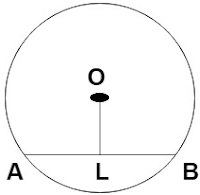a) AL > LB
b) AL < LB
c) AL ≠ LB
d) AL = LB

Question 12

If there are two separate circles drawn apart from each other, then the maximum number of common points they have:
a) 0
b) 1
c) 2
d) 3

Question 13

a) 5cm

b) 8cm
c) 4cm
d) 2cm

Question 14

The angle subtended by the diameter of a semi-circle is:

a) 90o
b) 45o
c) 180o
d) 60o

Explanation: Angle in a semi circle is always right

Question 15

Find the value of AB if radius of circle is 10cm and PQ = 12cm and RS = 16cm?

a) 14cm
b) 16cm
c) 4cm
d) 2cm

Question 16
If AB and CD are two chords of a circle intersecting at point E, as per the given figure. Then:

a) ∠BEQ > ∠CEQ
b) ∠BEQ = ∠CEQ
c) ∠BEQ < ∠CEQ
d) None of the above

Explanation:

OM = ON …………….. (Equal chords are always equidistant from the centre)

OE = OE  …………….. (Common)

OME = ONE …… (Each = 90o)

So, ΔOEM  ΔOEN ……… (by RHS congruence rule)

Hence, MEO = NEO …….. (by CPCT)

BEQ = CEQ

Question 17

a) 5cm

b) 8cm
c) 4cm
d) 20cm

Explanation:

Let r be the radius of circle.
Since perpendicular from centre to a chord bisects the chord,
AR = RS/2 = 3cm and BP = PQ/2 = 4cm
In ΔBOP, OBP = 90°

By Pythagoras theorem, OP2 = OB2 + BP2
r2 = x2 + (4)2
r2 = x2 + 16  ——————- (i)
Similarly, In ΔAOR, OAR = 90°

By Pythagoras theorem, OR2 = OA2 + AR2
r2 = (7 – x)2 + (3)2
r2 = (7 – x)2 + 9  ———————— (ii)
From equation i and ii, x2 + 16 = (7 – x)2 + 9
x2 + 16 = 72 – 2 x 7 x x + x2 + 9
2 x 7 x x = 49 – 16 + 9
x = 3
Substituting value of x in equation i, r2 = 32 + 16
r = 5cm.

Question 18
If a line intersects two concentric circles with centre O at A, B, C and D, then:
a) AB = CD
b) AB > CD
c) AB < CD
d) None of the above
Explanation: See the figure below:

From the above fig., OM  AD.

Therefore, AM = MD …….. (1)

Also, since OM  BC, OM bisects BC.

Therefore, BM = MC ……… (2)

From equation (1) and equation (2)

AM – BM = MD – MC

AB = CD

Question 19
Two circles of radius 13cm and 17cm intersect at A and B. What is the distance between the centres of the circles if AB = 24cm?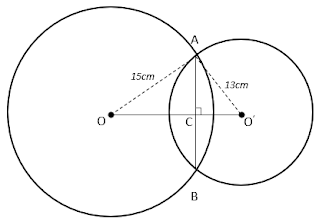a) 15cm
b) 25cm
c) 22cm
d) 14cm

Explanation:
Since line joining the centres of the circles bisects the common chord, AC = BC = 12cm.
Also, In ΔAOC, OCA = 90°

By Pythagoras theorem, OA2 = OC2 + AC2
152 = OC2 + 122
OC2 = 225 – 144
OC = 9cm
Similarly, In ΔAO’C, O’CA = 90°

By Pythagoras theorem, O’A2 = O’C2 + AC2
132 = O’C2 + 122
OC2 = 169 – 144
OC = 5cm
Now, OO’ = OC + O’C
OO’ = 9 + 5 = 14cm.

Question 20

In the below figure, the value of ∠ADC is:

Explanation: AOC = AOB + BOC

So, AOC = 60° + 30°

AOC = 90°

An angle subtended by an arc at the centre of the circle is twice the angle subtended by that arc at any point on the rest part of the circle.

= 1/2 × 90° = 45°

Question 21
In the given figure, find angle OPR.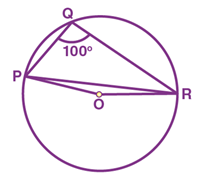a) 20°
b) 15°
c) 12°
d) 10°

Explanation: Major arc PR subtend Reflex POR at the centre of the circle and PQR in the remaining part of the circle

So, Reflex POR = 2 × PQR,

We know the values of angle PQR as 100°

So, Reflex POR = 2 × 100° = 200°

POR = 360° – 200° = 160°

Now, in ΔOPR,

OP and OR are the radii of the circle

So, OP = OR

Also, OPR = ORP = x

By angle sum property of triangle, we know:

POR + OPR + ORP = 180°

160° + x + x  = 180°

2x = 180° – 160°

2x = 20°  x = 10°

Question 22

a) 30º
b) 45º
c) 60º
d) 90º
Explanation:

Given that ∠AOB = 90º and ∠ABC = 30º
OA = OB (Radii of the circle)
Let ∠OAB = ∠OBA = x
In the triangle OAB,
∠OAB + ∠OBA + ∠AOB = 180° (By using the angle sum property of triangle)
⇒ x + x + 90° = 180°
⇒ 2x = 180° – 90°
⇒ x = 90°/ 2 = 45°
Therefore, ∠OAB = 45° and ∠OBA = 45°
By using the theorem, “ the angles subtended by arcs at the centre of the circle double the angle subtended at the remaining part of the circle”, we can write
∠AOB = 2∠ACB
This can also be written as,
∠ACB = ½ ∠AOB = (½) × 90° = 45°
Now, apply the angle sum property of triangle on the triangle ABC,
∠ACB + ∠BAC + ∠CBA = 180°
45° + 30° + ∠BAC = 180°
75° + ∠BAC = 180° ⇒ ∠BAC = 180° - 75°
⇒ ∠BAC = 105°
∠CAO + ∠OAB = 105°
∠CAO + 45° = 105°
⇒ ∠CAO = 105° - 45° = 60°
Question 23

ABCD is a cyclic quadrilateral such that AB is a diameter of the circle circumscribing it and ∠ADC = 140º, then ∠BAC is equal to: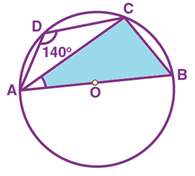a) 30º
b) 40º
c) 50º
d) 80º

Explanation:

We know that the sum of opposite angles of a cyclic quadrilateral is 180°.

Hence, ADC + ABC = 180°

140° + ABC = 180°

ABC = 180° – 140° = 40°

Now  ACB = 90° . . . . . . .  Angles in the semi circle is right

By using the angle sum property of triangle in the triangle, ABC,

CAB + ABC + ACB = 180°

CAB + 40° + 90° = 180°

CAB + 130°   = 180°

CAB = 180° – 130°

CAB = 50°

Therefore, CAB or BAC =50°.

Question 24

In the given figure, if ∠OAB = 40º, then ∠ACB is equal to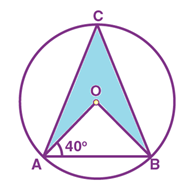a) 40º
b) 50º
c) 60º
d) 70º
Explanation:

Given that OAB = 40º,

In  the triangle OAB,

OAB = OBA  = 40°  …… ( angles opposite to equal sides are equal)

Now, by using the angle sum property of triangle, we can write

AOB + OBA + BAO = 180°

AOB + 40° + 40° = 180°

AOB = 180 – 80° = 100°

Since, the angle subtended by an arc at the centre is twice the angle subtended by it at the remaining part of the circle

AOB = 2 ACB

ACB = ½ AOB

Hence, ACB = 100°/2 = 50°.

Question 25

In the given figure, if ∠ABC = 20º, then ∠AOC is equal to: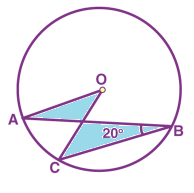a) 10º
b) 20º
c) 40º
d) 60º
Explanation:
Given that, ∠ABC = 20º.
since the angle subtended by an arc at the centre of the circle is double the angle subtended at the remaining part.
∠AOC = 2∠ABC
∠AOC = 2 × 20° = 40°.

Question 26
In the given figure, if OA = 5 cm, AB = 8 cm and OD is perpendicular to AB, then CD is equal to: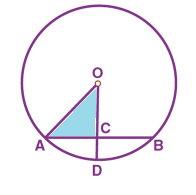a) 2 cm
b) 3 cm
c) 4 cm
d) 5 cm

Explanation:

From the given diagram, we can observe that OC is perpendicular to chord AB. Therefore, OC bisects the chord AB

Hence. AC = CB

Also,  AC + CB = AB

AC + CB = 8

2AC = 8 (Since, AC = CB)

AC = 8/2 = 4 cm

As, the triangle OCA is a right-angled triangle, by using Pythagoras theorem, we can write

AO2 = AC2 + OC2

52 = 42 + OC2

52 − 42 = OC2

OC2 = 9

OC = 3 cm

As, OD is the radius of the circle, OA = OD = 5cm

CD = OD - OC

CD = 5 - 3 = 2 cm

Hence, the value of CD is equal to 2cm.

Question 27

a) 90°
b) 45°
c) 60°
d) 20°

Explanation: Since ABCD is a cyclic quadrilateral, Sum of either pair of opposite angles of cyclic quadrilateral is 180°
1 + 2 = 180°
3k + 6k = 180°  k = 20°
Now, x = 1 [Exterior angle formed when one side of cyclic quadrilateral is produced is equal to the interior opposite angle]

x = 3k  x = 60°.

Question 28

If PQRS is a cyclic quadrilateral and PQ is diameter, find the value of ∠PQS.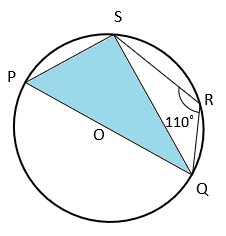a) 45°
b) 110°
c) 20°
d) 80°
Explanation: Since PQRS is a cyclic quadrilateral, Sum of either pair of opposite angles of cyclic quadrilateral is 180°.  QRS + QPS = 180°

QPS = 70°  ————–(i)
Also, PSQ = 90°  [Angle in a semicircle] ———-(ii)

In ΔPSQ,
PSQ + SPQ + SQP = 180°  [Angle sum property of triangle]

90° + 70° + PQS = 180°  [from equation i and ii]
PQS = 20°.

Question 29

In the given figure, BC is the diameter of the circle and ∠BAO = 60º. Then ∠ADC is equal to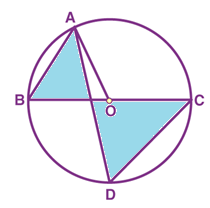a) 30º
b) 45º
c) 60º
d) 120º
Explanation:

Given that BAO = 60°

Since OA = OB, OBA = 60°

Then ADC = 60° ………. (Angles in the same segment of a circle are equal).

Question 30
If RS is equal to the radius of circle, find the value of ∠PTQ.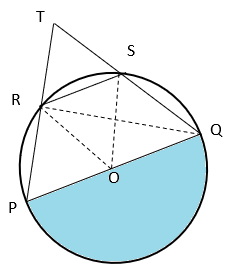a) 90°
b) 60°
c) 45°
d) 30°
Explanation: Join OS, OR and RQ
From figure, ∠PRQ = 90° as angle in a semicircle is right angle.
Now, ∠QRT = 180° – ∠PRQ  [Linear Pair]
⇒ ∠QRT = 180° – 90° = 90° ————–(i)
In ΔROS, OS = OR = RS ⇒ ΔROS is an equilateral triangle.
⇒ ∠ROS = 60°
Now, ∠SQR = ½ ∠ROS  [angle subtended by an arc of circle at the centre is twice the angle subtended by the arc on circumference]
⇒ ∠SQR = 30° —————-(ii)
In ΔTQR, ∠TRQ + ∠QTR + ∠TQR = 180°  [Angle sum property of triangle]
⇒ 90° + ∠PQR + 30° = 180°  [from equation i and ii]
⇒ ∠PQR = 60°.

Question 31

In the given figure, if ∠DAB = 60º, ∠ABD = 50º, then ∠ACB is equal to: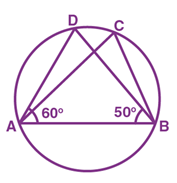a) 50º
b) 60º
c) 70º
d) 80º

Explanation:

DAB = 60º, ABD = 50º

By using the angle sum property in the triangle ABD

DAB + ABD + ADB = 180º

60º + 50º + ADB = 180º

ADB = ACB ……… (Angles in the same segment of a circle are equal).

ACB =70º

Question 32

In the given figure, if AOB is a diameter of the circle and AC = BC, then ∠CAB is equal to: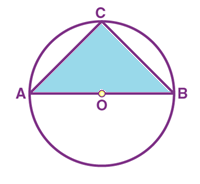a) 30º
b) 45º
c) 60º
d) 90º
Explanation:

We know that the angle in a semi circle is = 90°

Hence, ACB = 90°

Also, given that AC = BC

CAB = CBA = x …..  (let) (As, the angles opposite to equal sides are also equal)

Now, by using the angle sum property of triangle in ∆ACB, we can write

CAB + ABC + BCA = 180°

x + x + 90° = 180°

2x = 180° – 90°  2x = 90°  x = 45°

CAB = x = 45°

Question 33

a) 60°
b) 120°
c) 240°
d) 180°
Explanation: Reflex AOC = 240°

AOC = 360° – Reflex AOC = 360° – 240° = 120°
Since angle subtended by an arc of circle at the centre is twice the angle subtended by the arc on circumference, AOC = 2ABC

ABC = ½ AOC = 60°.

Question 34
If AB = 12 cm, BC = 16 cm and AB is perpendicular to BC, then the radius of the circle passing through the points A, B and C is: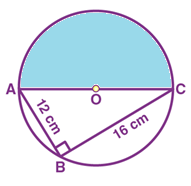a) 6 cm
b) 8 cm
c) 10 cm
d) 12 cm

Explanation:

Given that AB = 12 cm, BC = 16 cm and AB is perpendicular to BC.

Hence, AC is the diameter of the circle passing through points A, B and C.

Hence, ABC is a right-angled triangle.

Thus by using the Pythagoras theorem:

AC2 = (CB)2 + (AB)2

AC2 = (16)2 + (12)2

AC2 = 256 + 144

AC2 = 400

Hence, the diameter of the circle, AC = 20 cm.

Thus, the radius of the circle is 10 cm.

Question 35

a) 50°
b) 65°
c) 130°
d) 30°
Explanation: Join OP

In ΔPOQ, OP = OQ  [Radii of same circle]
OPQ = OQP = 20°  [Angles opposite to equal sides are equal]
In ΔPOR, OP = OR  [Radii of same circle]
OPR = ORP = 45°  [Angles opposite to equal sides are equal]
Now, QPR = OPR + OPQ = 45° + 20° = 65°

Angle subtended by an arc of circle at the centre is twice the angle subtended by the arc on circumference  ROQ = 2QPR = 2 x 65° = 130°.

Question 36

AD is the diameter of a circle and AB is a chord. If AD = 34 cm, AB = 30 cm, the distance of AB from the centre of the circle is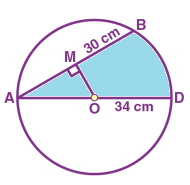a) 4 cm
b) 8 cm
c) 15 cm
d) 17 cm

Explanation:

Given that, Diameter, AD = 34 cm.

Chord, AB = 30 cm.

Hence, the radius of the circle, OA = 17 cm

Now, consider the figure.

From the centre “O”. OM is perpendicular to the chord AB.

(i.e) OM  AM

AM =  ½  AB

AM =  ½ (30) = 15 cm

Now by using the Pythagoras theorem in the right triangle AOM,

AO2 = OM2 + AM2

OM2 = AO2– AM2

OM2= 172 – 152

OM2 = 64

OM = √64

OM = 8 cm